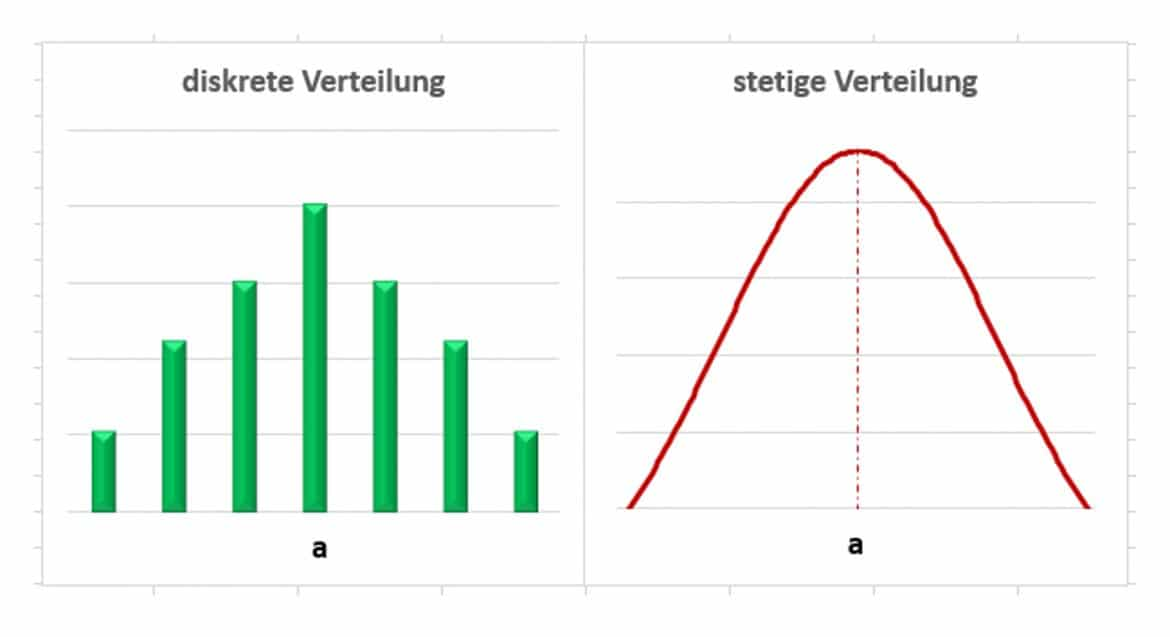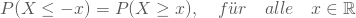# What is a perfectly symmetrical distribution

## Symmetrical distribution

A symmetric distribution is a special case of a probability distribution. More precisely, a distribution is called symmetric and a value a if the following equality holds for every realization x of the random variable X:### Graphic illustration of the symmetry property

The graphic on the left belongs to a discrete symmetrical distribution and shows its probability function. In contrast, the graphic on the right shows the density function of a continuous distribution. In both cases you can see their symmetry in the mirror-inverted course of the functions around the symmetry point a:If a takes the value zero, the distribution is symmetrical about the zero point and the following applies:You often look at distributions that are symmetrical about the expected value. Probably the most important example of a symmetrical distribution is the normal distribution, also known as the Gaussian distribution. But the uniform distribution or the Laplace distribution are also symmetrical, for example. Non-symmetrical continuous distributions are, for example, the log-normal distribution, the exponential distribution, the Weibull distribution, the gamma distribution, the beta distribution. Non-symmetrical discrete distributions are, for example, the multinomial distribution, the Poisson distribution, the geometric distribution or the hyptergeometric distribution. Some distributions have both symmetrical and non-symmetrical variants, such as the binomial distribution,